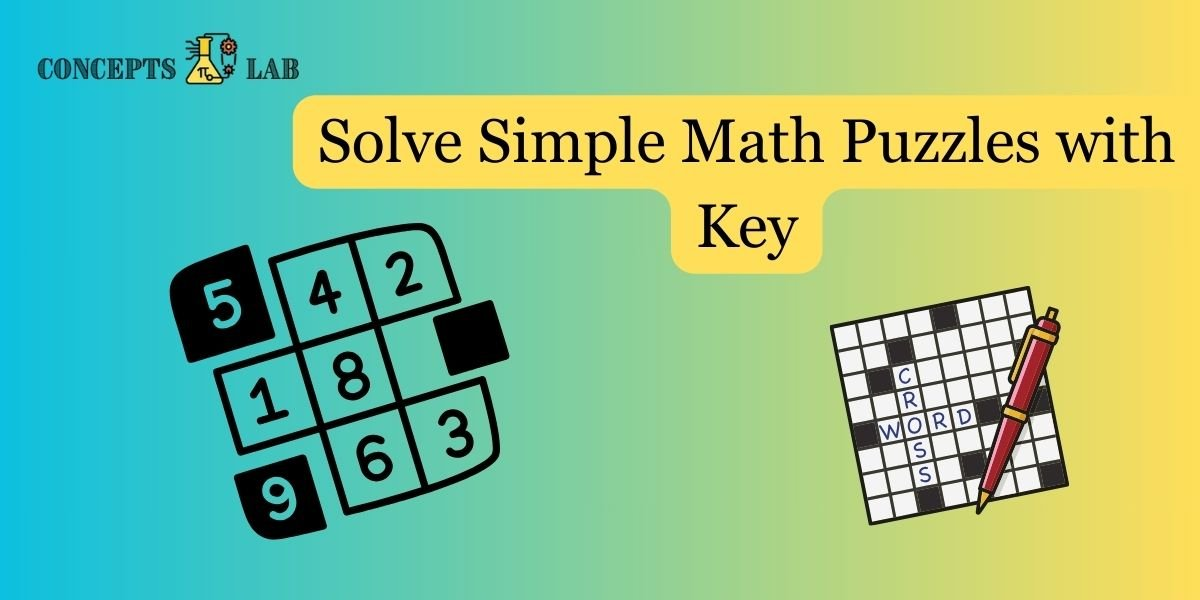### 1. Puzzle: The Missing Number

• 2, 4, 6, 8, __, 12, 14, __, 18

### 2. Puzzle: The Secret Code

• If A = 1, B = 2, C = 3, and so on, what does M + A + T + H equal?

### 3. Puzzle: The Magic Triangle

• In the magic triangle, each side’s sum is 9. Can you fill in the missing numbers
``````    5
/ \
/___\
3       6
``````

4. Puzzle: The 24 Challenge

Combine the numbers 4, 5, 6, and 7, using any operations (+, -, *, /) to make 24.

5. Puzzle: The Three Switches

• You are in a room with three light switches. Each switch corresponds to a different light bulb in the next room. You can only go into the next room once. How can you determine which switch corresponds to which bulb?

These puzzles cover a range of mathematical concepts, from numerical sequences to logic and problem-solving. Feel free to use them for fun and engaging math activities!

1. The missing numbers are 10 and 16. The sequence is counting by twos.
2. MATHEMATICS equals 13 + 1 + 20 + 8 + 5 + 13 + 1 + 20 + 9 + 3 + 19, which equals 100.
3. The missing numbers are 1 and 2. Each side of the triangle sums to 9 (5 + 3 + 1 and 5 + 2 + 6).
4. Combine the numbers 4, 5, 6, and 7, using any operations (+, -, *, /) to make 24.
5. Turn on the first switch and leave it on for a few minutes. Then turn it off and turn on the second switch. Enter the room. The bulb that is on corresponds to the switch left on. The bulb that is off and warm corresponds to the first switch, and the bulb that is off and cool corresponds to the third switch.